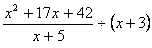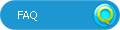# HotmathTitle:
Hotmath
Author:
Hotmath
Chapter: Rational Expressions and Rational Equations Section: Multiplying and Dividing Rational Expressions

Problem: 1

Multiply and then simplify the product.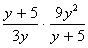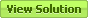Problem: 3

Multiply and then simplify the product.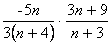Problem: 5

Multiply and then simplify the product.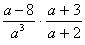Problem: 7

Multiply and then simplify the product.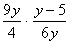Problem: 9

Multiply and then simplify the product.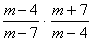Problem: 11

Multiply and then simplify the product.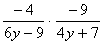Problem: 13

Multiply and then simplify the product.Problem: 15

Multiply and then simplify the product.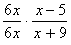Problem: 17

Multiply and then simplify the product.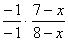Problem: 19

Multiply and then simplify the product.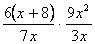Problem: 21

Multiply and then simplify the product.Problem: 23

Divide and simplify.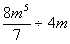Problem: 25

Divide and simplify.Problem: 27

Divide and simplify.Problem: 29

Divide and simplify.Problem: 31

Divide and simplify.Problem: 33

Divide and simplify.Problem: 35

Divide and simplify.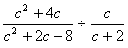Problem: 37

Divide and simplify.Problem: 39

Divide and simplify.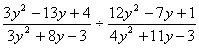Problem: 41

Divide and simplify.